post by Robert_AIZI · 2022-10-12T18:04:48.915Z · LW · GW · 2 comments

## Contents

  Background: What are Tensors?
The Algorithm Designing Game
How the AI Plays
How Impressed Should We Be?
Lessons for AI Safety
None


[Cross-posted from my Substack at https://aizi.substack.com/p/article-review-googles-alphatensor]How AlphaTensor sees matrices, probably

Last week, Google announced AlphaTensor, “the first artificial intelligence (AI) system for discovering novel, efficient, and provably correct algorithms for fundamental tasks such as matrix multiplication”. In this post, I want to rephrase their press release and Nature article in terms that make sense to me (and hopefully you, the reader), and share some thoughts on whether this is significant and holds any AI safety lessons.

## Background: What are Tensors?

Tensors are everywhere in our world: from press releases by Google, to Nature articles by Google, to AlphaTensor, the name of the agent trained by Google. But what are tensors?

Grids of numbers. Tensors are a grid of numbers. A tensor with two axes is a rectangular grid of numbers, a.k.a. a matrix, and a tensor with one axis is just a vector. Python programmers will be familiar with tensors as ndarrays. You can add tensors of the same size componentwise, and multiply them by scalars, so they form a vector space. You can make tensors more complicated if you want, but “grids of numbers” is all you need to know for this paper.

The goal of Google’s AI agent is to find fast algorithms for multiplication of matrices. I’ll pretend we’re working with just n-by-n square matrices, but the agents in the article are trained to find algorithms for n-by-m times m-by-p matrix multiplication for . Matrix multiplication is one of the most common operations performed by a computer, but it can be slow, especially for large matrices. The speed of these algorithms are measured in multiplications, since that makes up the bulk of the runtime for any of these algorithms (addition is relatively fast). The intro-to-linear-algebra method for matrix multiplication takes  multiplications to multiply n-by-n matrices, but its been known since the 1969 Strassen algorithm that you can save multiplications by doing more additions (Strassen’s original algorithm multiplies 2-by-2s in 7 multiplications instead of 8, and a variant called Strassen-square multiplies n-by-ns in roughly  multiplications).

So, what does matrix multiplication have to do with tensors? The first insight used in the paper is to think of matrix multiplication as a bilinear operator on , the set of n-by-n matrices. That is, matrix multiplication is a function  which is linear in the first component and the second component. And just as linear transformations can be uniquely encoded as matrices (2-axis tensors), bilinear transformations can be uniquely encoded as 3-axis tensors.

Stop. If that previous sentence makes sense to you, or you’re happy to accept it as a black box, skip the rest of the paragraph. Otherwise, here are the mathematical details of how:  as a vector space has a basis of size , consisting of the matrices  which are 0 everywhere except for a 1 in the (i,j)th component. Just as a linear transformation is determined by where it sends basis elements, bilinear transformations are determined by where they send pairs of basis elements, so a bilinear transformation g is uniquely determined by the values . Each  is itself a matrix in , so it can be uniquely written as a linear combination of the basis elements s. Thus for the  choices of  and the -choices of , we can uniquely represent  by the  numbers which are the coefficients of the . Putting those numbers in a logically-organized grid, we’d have a -by--by- grid of numbers, i.e. a 3-axis tensor! Alternatively, we can reshape that 3-axis tensor and think about it as a 6-axis n-by-n-by-n-by-n-by-n-by-n tensor, where the number in the (i, j, k, l, p, q)th position is the coefficient on the  term of .

You can do the steps above for any bilinear transformation, including matrix multiplication. It is straightforward to check that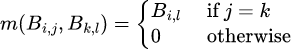so in the 6-axis tensor representation of multiplication, the entry in the (i, j, k, l, p, q)th entry is 1 if j = k, i = p, and q = l, and 0 otherwise.

This "coordinatization" of bilinear transformations is one of the key tricks of the article, and will form the basis of the Score Card Tensor below.

## The Algorithm Designing Game

AlphaTensor finds algorithms by a cleverly encoding “find an algorithm” as a game, such that finding moves to win the game is equivalent to finding an algorithm that multiplies matrices.

The game being played uses two tensors:

The Listed Steps Tensor is an -by-3-by-R tensor where R = 0 at the start of the game, and in each turn the player adds a -by-3-by-1 slice. At the end of the game, the -by-3-by-R tensor will describe an algorithm to do matrix multiplication with R multiplications.

The Score Card Tensor is an -by--by- tensor which starts as the coordinitization of matrix multiplication (in the process described above), and updates throughout the game based on the steps you add to the Listed Steps Tensor. The goal of the game is to zero out every entry on the Score Card Tensor.

These are my names for the tensors, but you can see both tensors in Figure 1.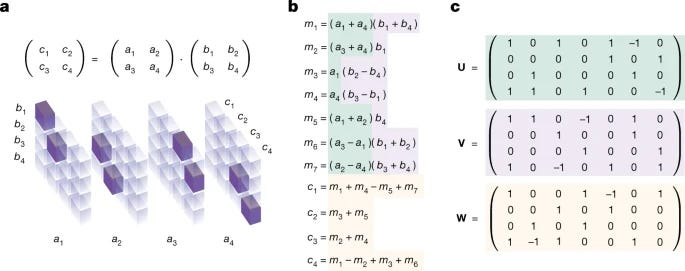Figure 1 from https://www.nature.com/articles/s41586-022-05172-4. Figure 1a shows the Score Card Tensor as it begins the game (i.e. the coordinitization of matrix multiplication), and Figure 1c shows the Listed Steps Tensor for Strassen algorithm shown in Figure 1b. Here, n=2 and R=7.

This encoding results in a 1-player game similar to the “solve a Rubik’s cube” game. There are a finite number of operations you can perform (steps described by -by-3-by-1 tensors/rotations of the cube faces), and each operation changes the underlying state (the Score Card Tensor/the pattern of the cube), you win once everything is orderly (Score Card Tensor is 0/all the colors are in the right spots), and your goal is to get there in as few moves as possible. However, the matrix multiplication game is harder because there are a staggering number of possible moves. Each move means choosing an -by-3-by-1 tensor, we’ll see there are 5 choices for each entry, so there are  possible moves at each stage! For n = 5, that’s more than  possible moves!

Now let’s explain how the Listed Steps Tensor encodes an algorithm, and how those steps change the Score Card Tensor.

The algorithm is:
Inputs: Two n-by-n matrices A and B, and -by-3-by-R Listed Steps Tensor.

Output: Matrix C. (For correct Listed Steps Tensor, C = AB.)

1. Initialize a length  vector c=0.
2. Reshape matrices A and B into length  vectors a and b, respectively.
3. Repeat these steps for all R of the -by-3-by-1 slices of Listed Steps Tensor.
1. Separate the -by-3-by-1 slice of the Listed Steps Tensor into three length  vectors u, v, and w.
1. Compute  (the dot product aka inner product aka scalar product).
2. Compute  (the dot product aka inner product aka scalar product).
3. Compute   (a number multiplication).
4. Update  .
4. Reshape c into an n-by-n matrix C.

Note that the only multiplications in this algorithm are in steps 3.2-3.5. A key insight is that by restricting the entries of the Listed Steps Tensor (and hence u, v, and w) to a well-chosen set F, we can make the multiplications in steps 3.2, 3.3, and 3.5 very fast. Therefore, the only “normal speed” multiplication in in step 3d. Since we loop over that step R times, if we have a correct Listed Steps Tensor, we have found a matrix multiplication algorithm that only uses R “normal speed” multiplications.

What set F can make your multiplications quick? The article uses , which makes sense because multiplying by 0 and 1 are fast operations, negation is a fast operation, and multiplying by 2 is fast for a binary computer (for integers you simply bitshift, and for floats you just increase the exponent by 1).

Next: How does a step update your Score Card Tensor, and how can you be sure that the algorithm will result in multiplication? Here we use our understanding of coordinitization. Each loop of Step 3 in our algorithm results in computing , which can be easily verified to be a bilinear form in a and b. Therefore, we can coordinatize the function , resulting in an -by--by- tensor  for step  between 1 and R. The overall transformation computed by the algorithm in the first r loops is . We define , our Score Card Tensor after the r-th step by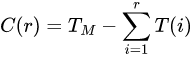where  is the tensor representation of matrix multiplication. Our goal is to end up with , in which case we can rearrange the equation and see that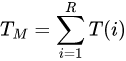which confirms that the algorithm correctly computes matrix multiplication since two bilinear forms are equal if and only if their coordinizations are equal.

To summarize, the overall structure of the game is:

1. Initialize the Score Card Tensor to be , and initialize the Listed Steps Tensor to be an -by-3-by-0 tensor.
2. On each turn:
1. The player chooses an -by-3-by-1 tensor with entries in F.
2. Update the Listed Steps Tensor by appending this new step.
3. Update the Score Card Tensor by subtracting off , the tensor corresponding to this bilinear transformation.
4. If the Score Card Tensor is all 0s, the player wins.
3. The player’s score is R, the length of the Listed Steps Tensor. The Listed Steps tensor describes a matrix multiplication algorithm using R multiplications, so a lower score is better.

So if we can just get an AI that chooses good tensors in step 2a, it will discover matrix multiplication algorithms for us. How does the AI do that?

## How the AI Plays

What the AI agent actually learns is how to score Score Card Tensors. Roughly speaking, it is trying to estimate the rank of a Score Card Tensor, which is the number of moves to win the game from that Score Card Tensor with optimal play. Once you have a good vibe-judging AI, here’s how the AI can play the game:

1. On each of its turns, it has a Score Card Tensor and needs to provide another algorithm step, described by an -by-3-by-1 tensor.
2. It randomly generates a number of possible algorithm steps (say 1000), and for each one it computes what the new Score Card Tensor would be if that was your next step.
3. For each of those 1000 new Score Card Tensors, estimate the rank of each.
4. Choose the step that minimizes the estimated rank of the modified Score Card Tensor.

I should confess that this is the part of my understanding that I feel shakiest on. At the very least, there are a few nuances to let things work better in practice:

• The game has a turn limit, and if the agent reaches the turn limit it gets a penalty based on the approximate rank of its final Score Card Tensor.
• In addition to the current Score Card Tensor, the AI’s rank estimator takes as input some previous number of Score Card Tensors, and some scalars (including the time stamp so it knows if its running out of time).
• Instead of deterministically choosing the rank-minimizing step, the agent may choose an option at random, weighting towards choices with lower ranks.
• The agent doesn’t just judge rank after one move, but looks ahead a few moves using Monte Carlo Tree Search.

Having just said all of that, I have a point of confusion, and I’m not sure if it’s my fault or the fault of the article. If the AI plays as I described, I’d expect it to trouble finding the final move that wins the game. Even with a perfect rank-estimation algorithm, the agent is still limited by only assessing the random options before it, and for the final move there’s essentially only one correct step to finish the game, so the agent is unlikely to finish the game. If the agent does have that problem, what we’d see is the agent will quickly decrease the rank of the Score Card Tensor but get stuck around rank 1, as shown in the diagram below.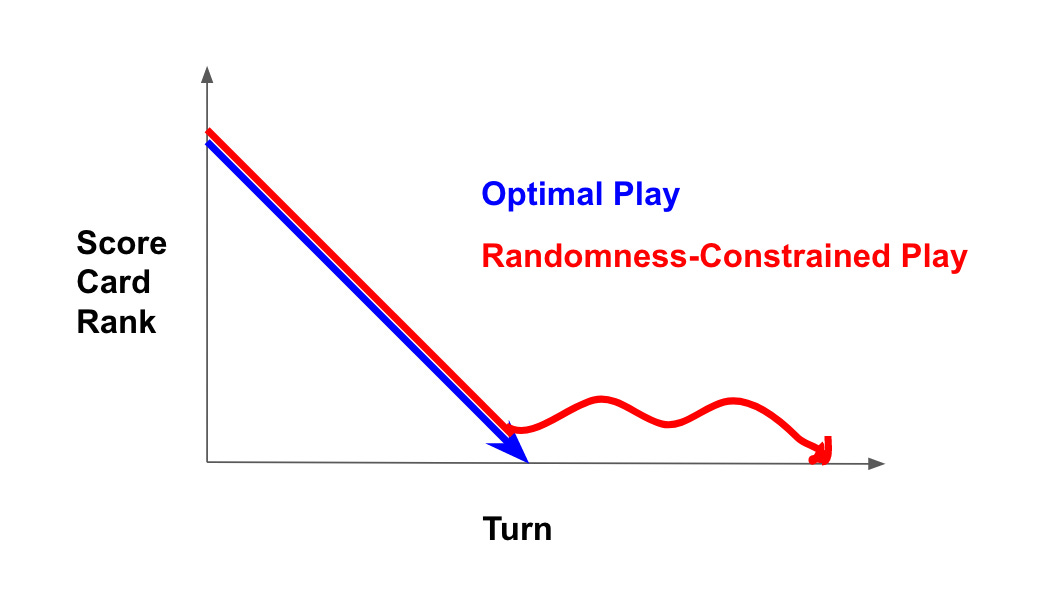Does AlphaTensor’s score over time look like the blue line or the red curve?

Am I misreading the paper? It describes the approach as “a sample-based MCTS search algorithm”, and “at inference time, K actions are sampled from the head”, which sounds like what I described. And algorithms that are constrained by randomness wouldn't prevent you from achieving the results in the article: if you have an otherwise-good agent that has a bad game because of its randomly-produced options, you can just run it over and over until it gets better luck.

But I wonder if you could get even better performance by incorporating a non-AI rule system once you get to low ranks. For example, it should be fast and easy to check if the Score Card Tensor is rank 1 before every turn. It’s rank 1 if the th entry is , which you should be able to quickly check by computing ratios between entries. Then if it is rank 1 you could find the u, v, and w vectors and override the agent to make that your move for the turn, winning you the game. It’s not clear to me if the problem I described above happens, if there is a workaround for this, or if they just power through it by playing the game over and over again, but this is definitely something I’d try!

Now that we understand what the authors did, let’s talk about implications.

## How Impressed Should We Be?

Not very? [Edit: I meant this to refer to the capabilities improvements displayed by AI, which to me seem much smaller than milestone accomplishments like GPT-3 and diffusion models. I don’t want to dismiss the human ingenuity to adapt existing AI to this problem.] There are two subquestions: did we advance matrix multiplication algorithms, and is it impressive an AI can do this at all?

On the former question, I find their Figures 3 and 5 to be instructive: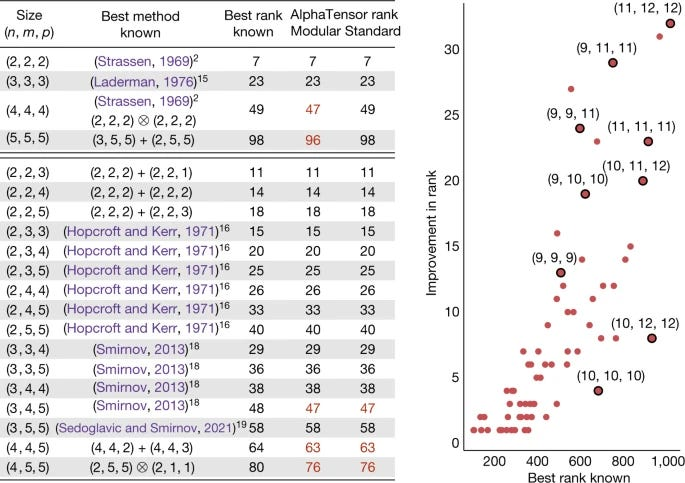Figure 3 from https://www.nature.com/articles/s41586-022-05172-4. Numbers highlighted in red are improves over existing algorithms.

You can see that in the most a priori interesting case (square, non-modular matrix multiplication) they did not make any improvements at all, and in cases where they did make improvements they reduced the rank just slightly. (Update: And less than a week later some authors improved on AlphaTensor’s 96 by finding a 95? Thanks to this tweet for alerting me.)

Figure 5 holds even more insights: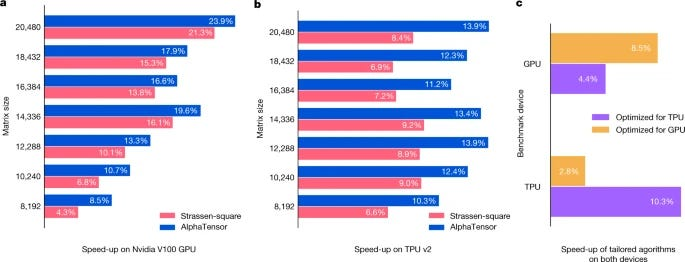Figure 5 from https://www.nature.com/articles/s41586-022-05172-4.

The first observation to make is that the headline “10-20% speed up” figure is relative to a baseline, and the speed up over Strassen-square (the old-but-fast algorithm) is much smaller, roughly in the 3-10% range. But hold on, why does the caption say “Speed-ups are measured relative to standard (for example, cuBLAS for the GPU) matrix multiplication”? If there’s already an industry standard that’s slower than Strassen-square, why isn’t Strassen-square the standard?

And thus we reach the galaxy brain insight: “speed isn’t everything”.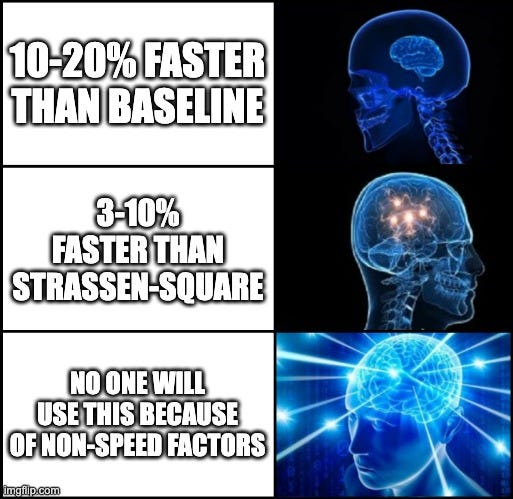In particular, wikipedia tells me Strassen-square has worse numerical stability, which I understand to be rather important. And the paper seems to be aware of this limitation: it meekly suggests someone else could build on their approach to “optimize metrics that we did not consider here, such as numerical stability or energy usage.”

Okay, so the speedups they promise aren’t huge or practical, but isn’t it impressive that an AI could design provably correct algorithms at all?

Yes and no. To me the key insight of the paper is to express matrix multiplication as a game, which is then subject to the optimizations of the AI agent. The authors (and those whose work they built on) deserve credit for this insight, but “we made a computer search this space” is a decades-old technique in mathematics. If this paper ends up shifting any paradigms, I think it will be shifting research questions from “how can we get an AI to do X?” to “we know an AI can do X if we can make it into a game, how do we do that?”.

Finally, what should we make of the “provably correct” claims that the press release mentions twice and the article four times? I’m underwhelmed. The algorithms are provably correct, in the same way that a number factorization is “provably correct” because you can multiply the factors and see that the product is correct. And the AI didn’t do any of the work to provide that proof. Instead, humans designed the rules of the game so that winning the game is equivalent to finding a provably correct matrix multiplication algorithm. It’s like claiming your chess-playing AI is “provably compliant with the rules of chess”. So I don’t think mathematicians need to worry about being obsoleted by AIs just yet.

## Lessons for AI Safety

At this point you’re probably asking: does this mean the robots will kill us all? I wish I knew. In the mean time, some tangentially related thoughts:

• This is yet another breakthrough that comes from humans finding the correct way to structure knowledge so that an AI agent can comprehend it. I want to make a full post assessing whether that’s how most or all capability advances happen, but the upshot would be that it’s harder to make predictions.
• In principle, this shows how AI takeoff could happen: AI finds a faster matrix multiplication algorithm, and since matrix multiplication is used in AI training, the AI can become smarter, making more discoveries and self-improving until it is arbitrarily powerful.
• In practice, this paper is not at all contributing to takeoff. The newly discovered algorithms are probably not going to be used for numerical stability reasons, and even if they were, a 10-20% speedup of one particular step is probably not going to translate into a much smarter AI.

1. ^

I am using “number of axes” instead of the sometimes-used terms “rank” or “dimension” to minimize confusion. In this post and the Nature article, the rank of a tensor is used to mean “the least number of simple tensors needed to sum to this tensor”.

2. ^

The technique is that if you want to multiply 4-by-4s, you treat each 4-by-4 matrix as a 2-by-2 of 2-by-2s which are susceptible to your 2-by-2 multiplication algorithm, using 7 lots of 7 multiplications, so you’ve multiplied 4-by-4s in 49 multiplications total. Repeating this trick gives you an algorithm for -by- for any k, and for other sizes you can just pad out the matrix with 0s. In general, this technique means any algorithm that takes R multiplications for m-by-ms lets you multiply all n-by-ns for n ≥ m in  multiplications.

3. ^

See this for a refresher.

4. ^

Pun intended.

5. ^

Recall that the inner product of two vectors  and  is .

6. ^

This is how I beat roguelikes.

7. ^

This is probably unfair, but I’m required by law to include a backronym.